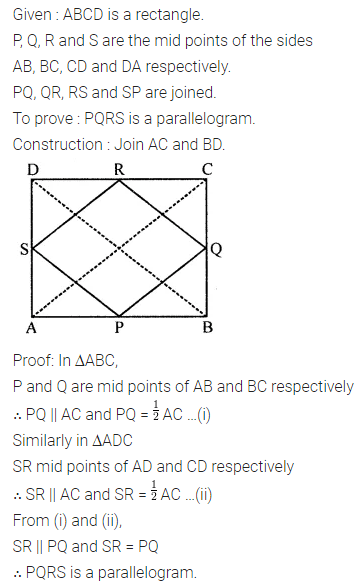# ML Aggarwal Class 8 Solutions for ICSE Maths Chapter 13 Understanding Quadrilaterals Objective Type Questions

## ML Aggarwal Class 8 Solutions for ICSE Maths Chapter 13 Understanding Quadrilaterals Objective Type Questions

Mental Maths
Question 1.
Fill in the blanks:
(i) The curves which have different beginning and endpoints are called …………
(ii) A curve which does not cross itself at any point is called a …………
(iii) A simple closed curve made up entirely of line segments is called a …………
(iv) A polygon in which each interior angle is less than 180° is called a …………
(v) 10 sided polygon is called …..
(vi) A polygon which has all its sides of equal length and all its angles of equal measure is called a …………
(vii) Sum of all exterior angles of a polygon is …………
(vi) A polygon which has all its sides of equal length and all its angles of equal measure is called a …………
(vii) Sum of all exterior angles of a polygon is …………
(viii) Sum of all interior angles of a n-sided polygon is …………
(ix) The adjacent angles of a parallelogram are …………
(x) If an angle of a parallelogram is a right angle, then it is called a …………
(xi) If two adjacent sides of a parallelogram are equal, then it is called a …………
(xii) It two adjacent sides of a rectangle are equal, then it is called a …………
(xiii) The diagonal of a rhombus bisect each other at …………
(xiv) A quadrilateral in which one pair of opposite sides is parallel is called a …………
(xv) A quadrilateral in which two pairs of adjacent sides are equal is called a …………
(xvi) If two non-parallel sides of a trapezium are equal then it is called …………
Solution: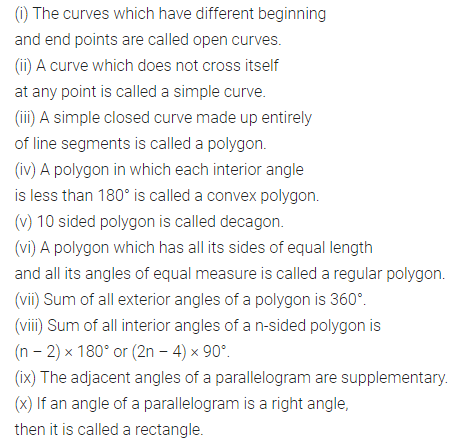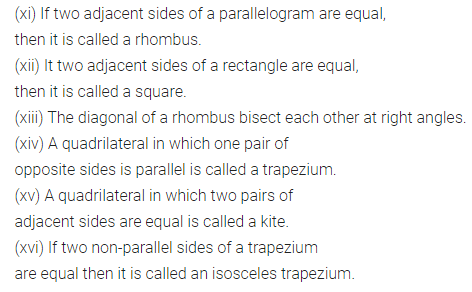Question 2.
State whether the following statements are true (T) or false (F):
(i) The curves which have the same beginning and endpoints are called open curves.
(ii) The region of the plane that lies inside the curve is called the interior of the curve.
(iii) A polygon in which at least one interior angle is greater than 180° is called convex polygon.
(iv) 6 sided polygon is called hexagon.
(v) Sum of all interior angles of a quadrilateral is 180°.
(vi) Each interior angle of a n-sided regular polygon is $$\frac{(2 n-4) \times 90^{\circ}}{n}$$.
(vii) The diagonals of a parallelogram bisect each other at right angles.
(viii) The opposite angles of a parallelogram are of equal measure.
(ix) The diagonals of a rhombus bisect the angles of a rhombus.
(x) The diagonals of a square are not equal.
(xi) Co-interior angles of a parallelogram are supplementary.
(xii) The diagonals of a kite bisect at right angles.
(xiii) All rectangles are squares.
(xiv) All rhombuses are parallelograms.
(xv) All squares are rhombuses and also rectangles.
(xvi) All squares are not parallelograms.
(xvii) All kites are rhombuses.
(xviii) All rhombuses are kites.
(xix) All parallelograms are trapeziums.
(xx) All squares are trapeziums.
Solution: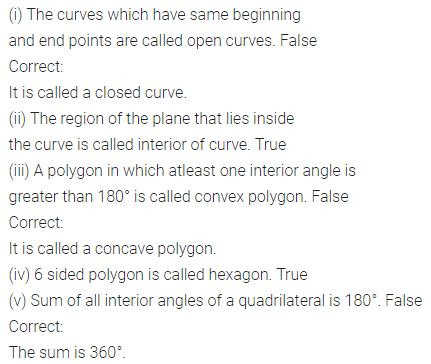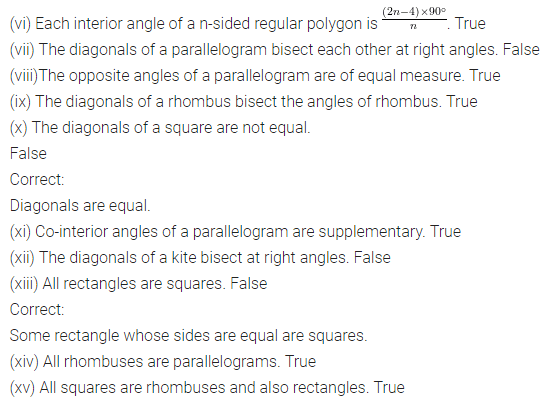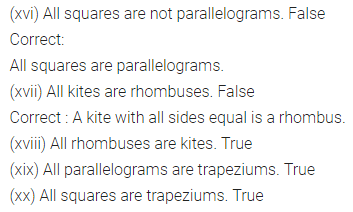Multiple Choice Questions
Choose the correct answer from the given four options (3 to 19):
Question 3.
Sum of all interior angles of an 11-sided polygon is
(a) 1620°
(b) 1440°
(c) 1260v
(d) none of these
Solution: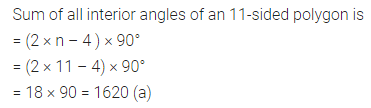Question 4.
If each interior angle of a regular polygon is 144°, then number of sides of polygon is
(a) 8
(b) 9
(c) 10
(d) 11
Solution: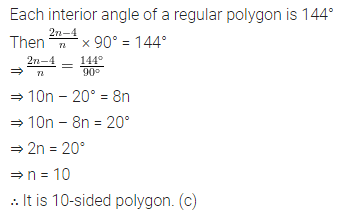Question 5.
If the sum of all interior angles of a polygon is 1260°, then number of sides of polygon is
(a) 6
(b) 7
(c) 8
(d) 9
Solution:Question 6.
The sum of all exterior angles of a pentagon is
(a) 590°
(b) 360°
(c) 180°
(d) none of these
Solution: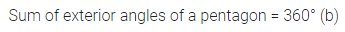Question 7.
If the ratio between an exterior and interior angle of a regular polygon is 1 : 5, then the number of sides of the polygon is
(a) 11
(b) 12
(c) 13
(d) 14
Solution:Question 8.
In the given figure, the value of x is
(a) 140°
(b) 50°
(c) 130°
(d) 40°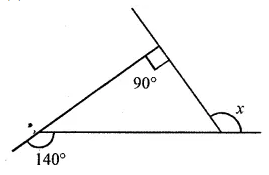Solution:Question 9.
In the given figure, the value of x is
(a) 120°
(b) 130°
(c) 140°
(d) 150°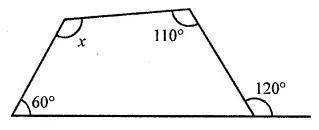Solution:Question 10.
In the given figure, the value of x + y + z + w is
(a) 180°
(b) 270°
(c) 300°
(d) 360°Solution:Question 11.
In the given figure, the value of x + y is
(a) 180°
(b) 190°
(c) 170°
(d) 160°Solution: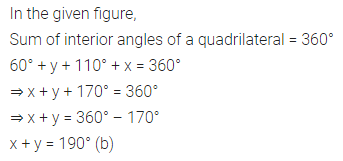Question 12.
The lengths of two adjacent sides of a parallelogram are in the ratio 1 : 2. If the perimeter of a parallelogram is 60 cm, then the length of its sides are
(a) 6 cm, 12 cm
(b) 8 cm, 16 cm
(c) 9 cm, 18 cm
(d) 10 cm, 20 cm
Solution: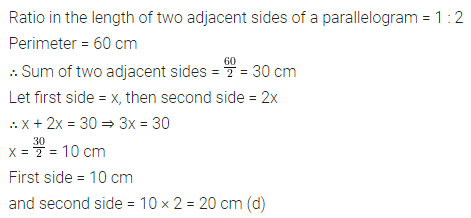Question 13.
In the given figure, ABCD is a parallelogram, the values of x and y respectively are
(a) 1 cm, 1 cm
(b) 2 cm, 1 cm
(c) 1 cm, 2 cm
(d) 2 cm, 2 cmSolution: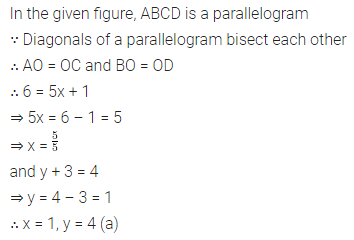Question 14.
In the given figure, ABCD is a parallelogram, the values of x, y and z respectively are
(a) 60°, 60°, 70°
(b) 60°, 70°, 60°
(c) 70°, 60°, 60°
(d) none of theseSolution: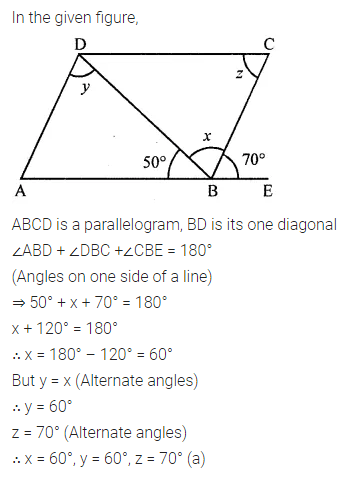Question 15.
In the given figure, ABCD is a rectangle, the value of angle x is
(a) 60°
(b) 90°
(c) 120°
(d) none of theseSolution: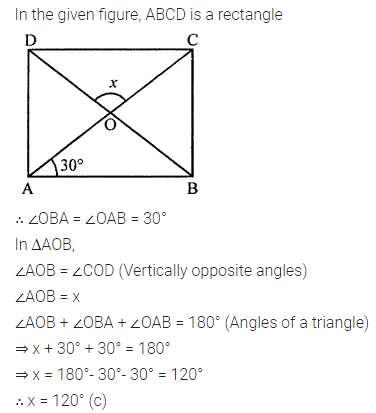Question 16.
In a rhombus ABCD, the diagonals AC and BD are respectively 8 cm and 6 cm. The length of each side of the rhombus is
(a) 7 cm
(b) 5 cm
(c) 6 cm
(d) 8 cm
Solution: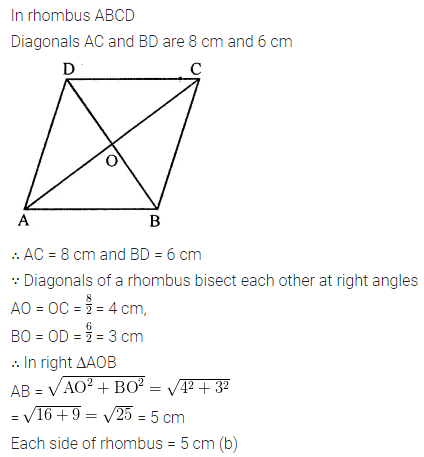Question 17.
In the given figure, ABCD is a square, the value of angle x is
(a) 30°
(b) 45°
(c) 60°
(d) not possible to find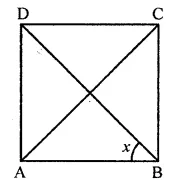Solution: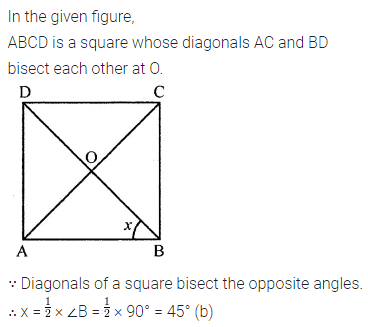Question 18.
In the given figure, ABCD is a kite, the value of angle x is
(a) 86°
(b) 100°
(c) 104°
(d) none of these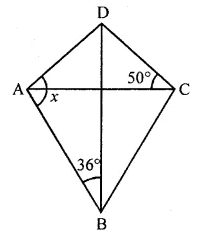Solution: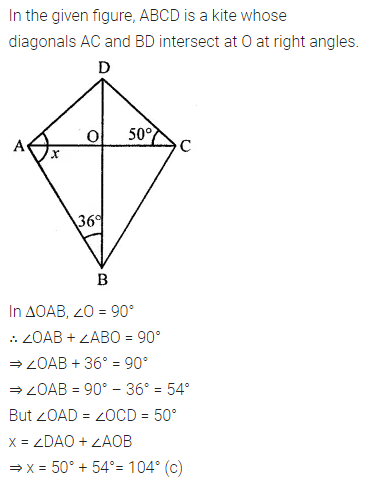Question 19.
In the given figure, ABCD is an isosceles trapezium. The values of x, y and z respectively are
(a) 110°, 110°, 70°
(b) 110°, 70°, 110°
(c) 70°, 110°, 110°
(d) none of these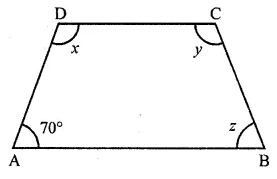Solution:Higher Order Thinking Skills (Hots)
Question 1.
In the given figure, ABCDEF is a regular hexagon. Prove that quadrilaterals ABDE and ACDF are parallelograms. Also prove that quadrilateral AGDH is a parallelogram.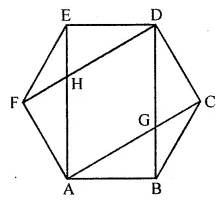Solution: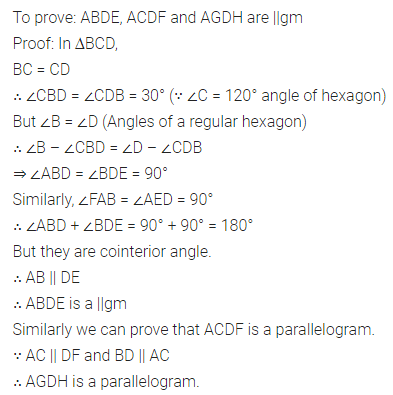Question 2.
In the given figure, ABCD is a parallelogram and M, N are the mid-points of sides BC, AD respectively. Prove that EA = AB = BF.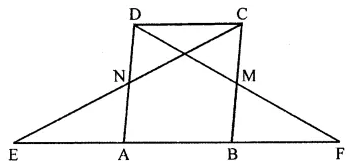Solution:Question 3.
Prove that the quadrilateral formed by joining the mid-points of the adjacent sides of a rectangle is a parallelogram.
Solution: